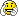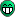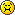# Histogram constractors-Histo from Ascii file

I am trying to create a histogram from an ascii file. My simple macro to do it, is

[code] #include “Riostream.h”
void HistoFromAscii(){

``````gROOT->SetStyle("Plain");
gStyle->SetOptStat(0000);
gStyle->SetOptFit(1111);
gStyle->SetOptTitle(0);

TCanvas *c = new TCanvas("c", "c");

TString dir = gSystem->UnixPathName(gInterpreter->GetCurrentMacroName());
dir.ReplaceAll("HistoFromAscii.C","");
dir.ReplaceAll("/./","/");
ifstream in;
in.open(Form("%sC6D62_e1405_m1.txt",dir.Data()));

Float_t x,y;
Int_t nlines = 0;
Int_t channels=3075;

//TH1F *h1 = new TH1F("h1","Total",channels,1,channels);//<--- This constractor is OK
TH1F *h1 = new TH1F();//<--- This constractor creates a weird histogram

while (1) {
in >> x >> y;
if (!in.good()) break;
if (nlines < 5) printf("x=%8f, y=%8f, nlines=%d\n",x,y,nlines);
h1->Fill(nlines,y);
nlines++;
}
printf("Found %d points\n",nlines);

in.close();

h1->SetLineColor(kRed);
h1->Draw();
//cout << "nlines = " << nlines << endl;
//h1->GetXaxis()->SetRangeUser(1,nlines);
``````

}[/code]

My ascii file is the following http://pastebin.com/0C7vyupb

When I use the constractor

``TH1F *h1 = new TH1F("h1","Total",channels,1,channels);``

I get the correct output which is something like that

If I use the constractor

``TH1F *h1 = new TH1F();``

I get the following histogram

which actually represents only the first bin. I tried to change the range, just in case, but this doesn’t seem to be the problem.

I think that the second constractor is the best, because the number of the total bins is not always the same. Any idea on what is going on? Does anyone know haw can this be solved?
Thank you very much in advance!

Note that I am running root while in an lxplus session.

Try:
TH1F *h1 = new TH1F()
h1->GetNbinsX()
h1->GetXaxis()->GetXmin()
h1->GetXaxis()->GetXmax()
delete h1
and then try:
int channels = 1000
TH1F *h1 = new TH1F(“h1”, “Total”, channels, 1, channels)
h1->GetNbinsX()
h1->GetXaxis()->GetXmin()
h1->GetXaxis()->GetXmax()
delete h1

BTW. If you don’t know the number of lines in advance, first scan your data file and count the lines, then create a “proper” histogram and scan this data file again filling this histogram.

P.S. Another good idea is to use a TGraph instead of a TH1F. Try simply:
TGraph *g1 = new TGraph(“C6D62_e1405_m1.txt”);
g1->Draw(“ALP”);

[quote=“Wile E. Coyote”]Try:
TH1F *h1 = new TH1F()
h1->GetNbinsX()
h1->GetXaxis()->GetXmin()
h1->GetXaxis()->GetXmax()
delete h1
and then try:
int channels = 1000
TH1F *h1 = new TH1F(“h1”, “Total”, channels, 1, channels)
h1->GetNbinsX()
h1->GetXaxis()->GetXmin()
h1->GetXaxis()->GetXmax()
delete h1

BTW. If you don’t know the number of lines in advance, first scan your data file and count the lines, then create a “proper” histogram and scan this data file again filling this histogram.

P.S. Another good idea is: use a TGraph instead of a TH1F:
TGraph *g1 = new TGraph(“C6D62_e1405_m1.txt”);
g1->Draw(“ALP”);[/quote]

I don’t think I understand, though. Your suggestion is to create the histogram, to get the bins, the minimum and maximum and then delete the histogram? If this is the case, I don’t understand why to do it.

It’s a good idea to loop over the ascii file and then create the histogram, but I think it will take much more time to perform 2 loops, than one, that’s I am trying to create the histogram using the second constractor.

I also want to avoid TGraph, beacause I want to use Histogram functions.

Run the commands, that I gave you, in a standard ROOT prompt and analyze the numbers that it printed.

I run the commands you gave and the output is the following

```root  h1->GetNbinsX() (const Int_t)1 root  h1->GetXaxis()->GetXmin() (const Double_t)0.00000000000000000e+00 root  h1->GetXaxis()->GetXmax() (const Double_t)2.00000000000000000e+03 root  delete h1 root  int channels = 1000 root  TH1F *h1 = new TH1F("h1", "Total", channels, 1, channels) root  h1->GetNbinsX() (const Int_t)1000 root  h1->GetXaxis()->GetXmin() (const Double_t)1.00000000000000000e+00 root  h1->GetXaxis()->GetXmax() (const Double_t)1.00000000000000000e+03 ```And now just “analyze” these numbers -> pay special attention to what “GetNbinsX” returned in both cases.BTW. I think I’ve already been correcting it so many times in the last years … but here’s the same correction again … TH1F(“h1”, “Total”, channels, 1, (channels + 1)) …I know that I get only the first bin printed;it’s in my first post.
The thing is that I don’t why and for certain I don’t know how to make it work properly!

You do NOT just “get only the first bin printed”. This histogram has ONE SINGLE bin only at all.Oh, I see…
And how can I fix it using this constractor?
Do you have any idea or advice?